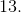# Common Core: 1st Grade Math : Numbers 10, 20, 30, 40, 50, 60, 70, 80, 90 Refer to One, Two, Three, Four, Five, Six, Seven, Eight, or Nine Tens: CCSS.MATH.CONTENT.1.NBT.B.2.C

## Example Questions

← Previous 1

### Example Question #1101 : Common Core Math: Grade 1

How many tens are in the number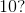Two

One

Five

Ten

Six

One

Explanation:

There is ain the tens place which means there is one ten in the number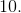### Example Question #1 : Numbers 10, 20, 30, 40, 50, 60, 70, 80, 90 Refer To One, Two, Three, Four, Five, Six, Seven, Eight, Or Nine Tens: Ccss.Math.Content.1.Nbt.B.2.C

How many tens are in the number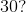Five

Thirty

Four

Thirteen

Three

Three

Explanation:

There is ain the tens place which means there are three tens in the number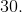### Example Question #2 : Numbers 10, 20, 30, 40, 50, 60, 70, 80, 90 Refer To One, Two, Three, Four, Five, Six, Seven, Eight, Or Nine Tens: Ccss.Math.Content.1.Nbt.B.2.C

How many tens are in the number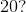Twenty

Three

Twelve

Two

One

Two

Explanation:

There is ain the tens place which means there are two tens in the number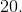### Example Question #3 : Numbers 10, 20, 30, 40, 50, 60, 70, 80, 90 Refer To One, Two, Three, Four, Five, Six, Seven, Eight, Or Nine Tens: Ccss.Math.Content.1.Nbt.B.2.C

How many tens are in the number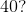Forty

Four

Five

Six

Fourteen

Four

Explanation:

There is ain the tens place which means there are four tens in the number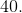### Example Question #4282 : Ssat Elementary Level Quantitative (Math)

How many tens are in the number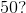Six

Three

Five

Seven

Fifty

Five

Explanation:

There is ain the tens place which means there are five tens in the number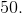### Example Question #6 : Numbers 10, 20, 30, 40, 50, 60, 70, 80, 90 Refer To One, Two, Three, Four, Five, Six, Seven, Eight, Or Nine Tens: Ccss.Math.Content.1.Nbt.B.2.C

How many tens are in the number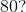Six

Four

Eight

Eighty

Sixty

Eight

Explanation:

There is ain the tens place which means there are eight tens in the number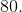### Example Question #2 : Numbers 10, 20, 30, 40, 50, 60, 70, 80, 90 Refer To One, Two, Three, Four, Five, Six, Seven, Eight, Or Nine Tens: Ccss.Math.Content.1.Nbt.B.2.C

How many tens are in the number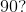Nine

Four

Ninty

Three

Forty

Nine

Explanation:

There is ain the tens place which means there are nine tens in the number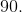### Example Question #4 : Numbers 10, 20, 30, 40, 50, 60, 70, 80, 90 Refer To One, Two, Three, Four, Five, Six, Seven, Eight, Or Nine Tens: Ccss.Math.Content.1.Nbt.B.2.C

How many tens are in the number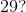Two

Twenty

Nine

Twenty-nine

Three

Two

Explanation:

There is ain the tens place which means there are two tens in the number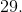### Example Question #1 : Numbers 10, 20, 30, 40, 50, 60, 70, 80, 90 Refer To One, Two, Three, Four, Five, Six, Seven, Eight, Or Nine Tens: Ccss.Math.Content.1.Nbt.B.2.C

How many tens are in the number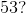Five

Thirty

Fifty

Three

Fifty-three

Five

Explanation:

There is ain the tens place which means there are five tens in the number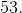### Example Question #10 : Numbers 10, 20, 30, 40, 50, 60, 70, 80, 90 Refer To One, Two, Three, Four, Five, Six, Seven, Eight, Or Nine Tens: Ccss.Math.Content.1.Nbt.B.2.C

How many tens are in the number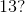Three

Thirteen

One

Ten

Three

There is ain the tens place which means there is one ten in the number